# Moontower on Gamma

The first option greek people learn after delta is gamma. Recall that delta represents how much an option’s price changes with respect to share price. That makes it a convenient hedge ratio. It tells you the share equivalent position of your option position. So if an option has a .50 delta, its price changes by \$.50 for a \$1.00 change in the stock price. Calls have positive deltas and puts have negative deltas (ie puts go down in value as the stock price increases). If you are long a .50 delta call option and want to be hedged, you must be short 50 shares of the stock (options refer to 100 shares of underlying stock). For small moves in the stock, your call and share position p/l’s will offset because you are “delta neutral”.

This is true for small moves only. “Small” is a bit wishy-washy because small depends on volatility and this post is staying away from that much complexity. Instead, we want to focus on how your delta changes as the stock moves. This is vital because if our option delta changes then your equivalent share position changes. If your position size changes, then that same \$1 move in the stock leads means your p/l changes are not constant for every \$1 change. If I’m long 50 shares of a stock, I make the same amount of money for each \$1 change. But if I’m long 50 shares equivalent by owning a .50 delta option, then as the stock increases my delta increases as the option becomes more in-the-money. That means the next \$1 change in the stock, produces \$60 of p/l instead of just \$50. We know that deep in-the-money options have a 1.00 delta meaning they act just like the stock (imagine a 10 strike call expiring tomorrow when the stock is trading for \$40. The option price and stock price will move perfectly in lockstep. The option has 100% sensitivity to the change).

A call option can go from .50 delta to 1.00 delta. Gamma is the change in delta for the change in stock. Suppose you own a .50 delta call and the stock goes up by \$1. The call is solidly in-the-money and perhaps its new delta is .60. That change in delta from .50 to .60 for a \$1 move is known as gamma. In this case, we say the option has .10 gamma per \$1. So if the stock goes up \$1, the delta goes up by .10.

While this is mechanically straightforward, some of the lingo around gamma is confusing. People spout phrases like “a squared term”, “curvature”, “convexity”. I’ve written about what convexity is and isn’t because I’ve seen it trip up people who should know better. See Where Does Convexity Come From?. In this post, we will demystify the relationship of these words to “gamma”. In the process, you will deeply improve your understanding of options’ non-linear nature.

How the post is laid out:

Explanations

• Acceleration
• The squared aspect of gamma
• Dollar gamma

Applications

• Constant gamma
• Strikeless products
• How gamma scales with price and volatility
• Gamma weighting relative value trades

# Explanations

## Acceleration

You already understand “curvature”. I’ll prove it to you.

You wake up tomorrow morning and see a bizarre invention in your driveway. An automobile with an unrivaled top speed.  You take it on an abandoned road to test it out. Weirdly, it accelerates slowly for a racecar. Conveniently for me, it makes the charts I’m about to show you easy to read.

You are traveling at 60 mph.

Imagine 2 scenarios:

1. You maintain that constant speed.
2. You accelerate such that after 1 minute you are now traveling at 80 mph. Assume your acceleration is smooth. That means over the 60 seconds it takes to reach 80 mph, your speed increases equally every second. So after 3 seconds, you are traveling 61 mph, at 6 seconds you are moving 62 mph. Eventually at 60 seconds, you are traveling 80 mph.

Graphically:In the acceleration case, what was your average speed or velocity during that minute?

Since the acceleration was smooth, the answer is 70 mph.

How far did you travel in each case?

Constant velocity: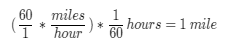Accelerate at 20mph per minute:If the acceleration is smooth, we can take the average velocity over the duration and multiply it by the duration to compute the distance traveled.

Let’s now continue accelerating this supercar by a marginal 20mph rate for the next 15 minutes and see how far we travel. Compare this to a vehicle that maintains 60 mph for the whole trip. The table uses the same logic — the average speed for the last minute assumes a constant acceleration rate.Let’s zoom in on the cumulative distance traveled at each minute:We found it! Curvature.

Curvature is the adjustment to the linear estimate of distance traveled that we would have presumed if we assumed our initial speed was constant. Let’s map this analogy to options.

• Time –> stock price

How much time has elapsed from T₀ maps to “how far has the stock moved from our entry?”

• Velocity –> delta

Delta is the instantaneous slope of the p/l with respect to stock price, just as velocity is the instantaneous speed of the car.

• Acceleration –> gamma

Acceleration is the change in our velocity just as gamma is the change in delta.

• Cumulative distance traveled –> cumulative p/l

Distance = velocity x time. Since the velocity changes, multiply the average velocity by time. In this case, we can double-check our answer by looking at the table. We traveled 52.5 miles in 15 minutes or 210 mph on average. That corresponds to our speed at the midpoint of the journey — minute 8 out of 15.
P/l = average position size x change in stock price. Just as our speed was changing, our position size was changing!

Delta is the slope of your p/l. That’s how I think about position sizes. Convexity is non-linear p/l that results from your position size varying. Gamma mechanically alters your position size as the stock moves around.

The calculus that people associate with options is simply the continuous expression of these same ideas. We just worked through them step-wise, minute by minute taking discrete averages for discrete periods.

## Intuition For the Squared Aspect Of Gamma

Delta is familiar to everyone because it exists in all linear instruments. A stock is a linear instrument. If you own 100 shares and it goes up \$1, you make \$100. If it goes up \$10, you make \$1,000. The position size is weighted by 1.00 delta (in fact bank desks that trade ETFs and stocks without options are known as “Delta 1 desks”).  Since you just multiply by 1, the position size is the delta. If you’re long 1,000 shares of BP, I say “you’re long 1,000 BP deltas”. This allows you to combine share positions and option positions with a common language. If any of the deltas come from options that’s critical information since we know gamma will change the delta as the stock moves.

If your 1,000 BP deltas come from:

500 shares of stock

+

10 .50 delta calls

that’s important to know. Still, for a quick summary of your position you often just want to know your net delta just to have an idea of what your p/l will be for small moves.

If you have options, that delta will not predict your p/l accurately for larger moves. We saw that acceleration curved the total distance traveled. The longer you travel the larger the “curvature adjustment” from a linear extrapolation of the initial speed. Likewise, the gamma from options will curve your p/l from your initial net delta, and that curvature grows the further the stock moves.

If you have 1,000 BP deltas all coming from shares, estimating p/l for a \$2 rally is easy — you expect to make \$2,000.

What if your 1,000 BP deltas all come from options? We need to estimate a non-linear p/l because we have gamma.

Let’s take an example from the OIC calculator.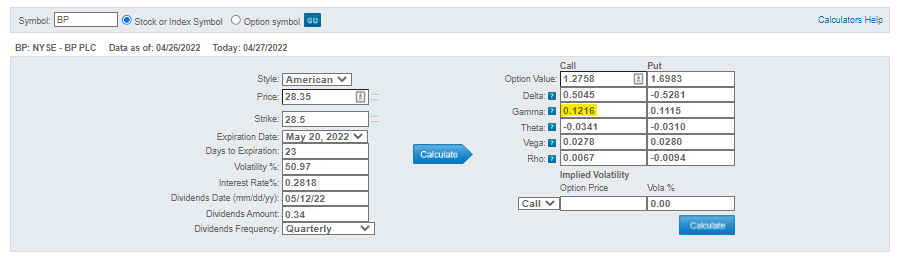The stock is \$28.35

This is the 28.5 strike call with 23 days to expiry. It’s basically at-the-money.

It has a .50 delta and .12 of gamma. Let’s accept the call value of \$1.28 as fair value.

Here’s the setup:

Initial position = 20 call options.

• Delta  =  1,000

.50 x 20 contracts x 100 share multiplier

• Gamma =  240

.12 x 20 contracts x 100 share multiplier

(the other greeks are not in focus for this post)

The greeks describe your exposures. If you simply owned 1,000 shares of BP you know the slope of your p/l per \$1 move…it’s \$1,000. That slope won’t change.

After \$1 rally:

• New delta = 1,240 deltas

.62 x 20 contracts x 100 share multiplier

Remember that gamma is the change in delta per \$1 move. That tells us if the stock goes up \$1, this call will increase .12 deltas, taking it from a .50 delta call to a .62 delta call.

That’s fun. As the stock went up, your share equivalent position went from 1,000 to 1,240.

Can you see how to compute your p/l by analogizing from the accelerating car example?

[It’s worth trying on your own before continuing]

### Computing P/L When You Have Gamma

(It’s ok to assume gamma is smooth over this move just as we said the acceleration was smooth for the car.)

Your average delta over the move = 1,120

1,120 x \$1 = \$1,120

You earned an extra \$120 vs a basic share position for the same \$1 move. That \$120 of extra profit is curvature from a simple extrapolation of delta p/l. Since that curvature is due to gamma it’s best to decompose the p/l into a delta portion and a gamma portion.

• The delta portion is the linear estimate of p/l = initial delta of 1,000 x \$1 = \$1,000
• The gamma portion of the p/l is the same computation as the acceleration example:

Your gamma represents the change in delta over the whole move. That’s 240 deltas of change per \$1. So on average, your delta was higher by 120 over the move. So we scale the gamma by the move size and divide by 2. That represents our average change in delta which we multiply by the move size to compute a “gamma p/l”.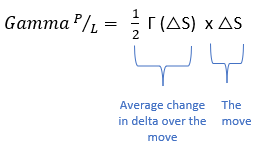where:

Γ = position weighted gamma = gamma per contract  x  qty of contracts  x  100 multiplier

△S = change in stock price

We can re-write this to make the non-linearity obvious — gamma p/l is proportional to the square of the stock move!## Generalizing Gamma: Dollar Gamma

In investing, we normally don’t speak about our delta or equivalent share position. If I own 1,000 shares of a \$500 stock that is very different than 1,000 shares of a \$20 stock. Instead, we speak about dollar notional. Those would be \$500,000 vs \$20,000 respectively. Dollar notional or gross exposures are common ways to denote position size. Option and derivative traders do the same thing. Instead of just referring to their delta or share equivalent position, they refer to their “dollar delta”. It’s identical to dollar notional, but preserves the “delta” vocabulary.

It is natural to compute a “delta 1%” which describes our p/l per 1% move in the underlying.For the BP example:

• Initial dollar delta = delta x stock price = 1,000 x \$28.35 = \$28,350 dollar deltas
• Δ1% = \$28,350/100 = \$283.50

You earn \$283.50 for every 1% BP goes up.

Gamma has analogous concepts. Thus far we have defined gamma in the way option models define it — change in delta per \$1 move. We want to generalize gamma calculations to also deal in percentages. Let’s derive dollar gamma continuing with the BP example.

1. Gamma 1%

Gamma per \$1 = 240

Of course, a \$1 move in BP is over 3.5% (\$1/\$28.35). To scale this to “gamma per 1%” we multiply the gamma by 28.35/100 which is intuitive.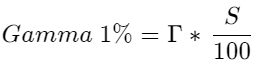Gamma 1% = 240 * .2835 = 68.04

So for a 1% increase in BP, your delta gets longer by 68.04 shares.

2. Dollar gamma

Converting gamma 1% to dollar gamma is simple. Just multiply by the share price.By substituting for gamma 1% from the above step, we arrive at the classic dollar gamma formula: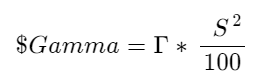Let’s use BP numbers.

\$Gamma = 240 * 28.35² / 100 = \$1,929

The interpretation:

A 1% rally in BP, leads to an increase of 1,929 notional dollars of BP due to gamma.

Instead of speaking of how much our delta (equivalent share position) changes, you can multiply dollar gamma by percent changes to compute changes in our dollar delta.

### Generalizing Gamma P/L For Percent Changes

In this section, we will estimate gamma p/l for percent changes instead of \$1 changes. Let’s look at 2 ways.

The Accelerating Car Method

The logic flows as follows (again, using the BP example):

• If a 1% rally leads to an increase of \$1,929 of BP exposure then, assuming gamma is smooth, a 3.5% rally (or \$1) will lead to an increase of \$6,751 of BP length because 3.5%/1% * \$1,929
• Therefore the average length over the move is \$3,375 (ie .5 * \$6,751) due to gamma
• \$3,375 * 3.5% = \$118 (This is very close to the \$120 estimate we computed with the original gamma p/l formula. This makes sense since we followed the same logci…multiply the average position size due to gamma times the move size.)

The Algebraic Method

We can adapt the original gamma p/l formula for percent changes.

We start with a simple identity. To turn a price change into a percent we simply divide by the stock price. If a \$50 stock increased \$1 it increased 2%If we substitute the percent change in the stock for the change in the stock we must balance the identity by multiplying by :We can double-check that this works with our BP example. Recall that the initial stock price is \$28.35: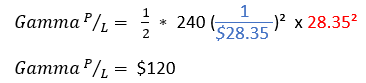This also checks out with the gamma p/l we computed earlier.

# Applications

### Constant Gamma

In all the explanations, we assume gamma is smooth or constant over a range of prices. This is not true in practice. Option gammas peak near the ATM strike. Gamma falls to zero as the option goes deep ITM or deep OTM. When you manage an option book, you can sum your positive or negative gammas across all your inventory to arrive at a cumulative gamma. The gamma of your net position falls as you move away from your longs and can flip negative as you approach shorts. This means gamma p/l estimates are rarely correct, because gamma calculations themselves are instantaneous. As soon as the stock moves, time passes, or vols change your gamma is growing or shrinking.

This is one of the most underappreciated aspects vol trading for novices. Vanilla options despite being called vanilla are diabolical because of path dependence. If you buy a straddle for 24% vol and vol realizes 30% there’s no guarantee you make money. If the stock makes large moves with a lot of time to expiration or when the straddle is not ATM then those moves will get multiplied by relatively low amounts of dollar gamma. If the underlying grinds to a halt as you approach expiration, especially if it’s near your long strikes, you will erode quickly with little hope of scalping your deltas.

Skew and the correlation of realized vol with spot introduce distributional effects to vol trading and may give clues to the nature of path dependence. As a trader gains more experience, they move from thinking simply in terms of comparing implied to realized vol, but trying to understand what the flows tell us about the path and distribution. The wisdom that emerges after years of trading a dynamically hedged book is that the bulk of your position-based p/l (as opposed to trading or market-making) will come from a simple observation: were you short options where the stock expired and long where it didn’t?

That’s why “it’ll never get there” is not a reason to sell options. If you hold deltas against positions, you often want to own the options where the stock ain’t going and vice versa. This starts to really sink in around year 10 of options trading.

### Strikeless Products

The path-dependant nature of vanilla options makes speculating on realized vol frustrating. Variance swaps are the most popular form of “strikeless” derivatives that have emerged to give investors a way to bet on realized vols without worrying about path dependence. Conceptually, they are straightforward. If you buy a 1-year variance swap implying 22% vol, then any day that the realized vol exceeds 22% you accrue profits and vice versa(sort of1). The details are not important for our purpose, but we can use what we learned about gamma to appreciate their construction.

A derivative market cannot typically thrive if there is no replicating portfolio of vanilla products that the manufacturer of the derivative can acquire to hedge its risk. So if variance swaps exist, it must mean there is a replicating portfolio that gives a user a pure exposure to realized vol. The key insight is that the product must maintain a fairly constant gamma over a wide range of strikes to deliver that exposure. Let’s look at the dollar gamma formula once again.We can see that gamma is proportional to the square of the stock price. While the gamma of an option depends on volatility and time to expiration, the higher the strike price the higher the “peak gamma”. Variance swaps weight a strip of options across a wide range of strikes in an attempt to maintain a smooth exposure to realized variance. Because higher strike options have a larger “peak” gamma, a common way to replicate the variance swap is to overweight lower strikes to compensate for their smaller peak gammas. The following demonstrates the smoothness of the gamma profile under different weighting schemes.

Examples of weightings:

Constant = The replicating strip holds the same number of 50 strike and 100 strike options

1/K = The replicating strip holds 2x as many 50 strike options vs 100 strike

1/K² = The replicating stripe holds 4x as many 50 strike options vs 100 strike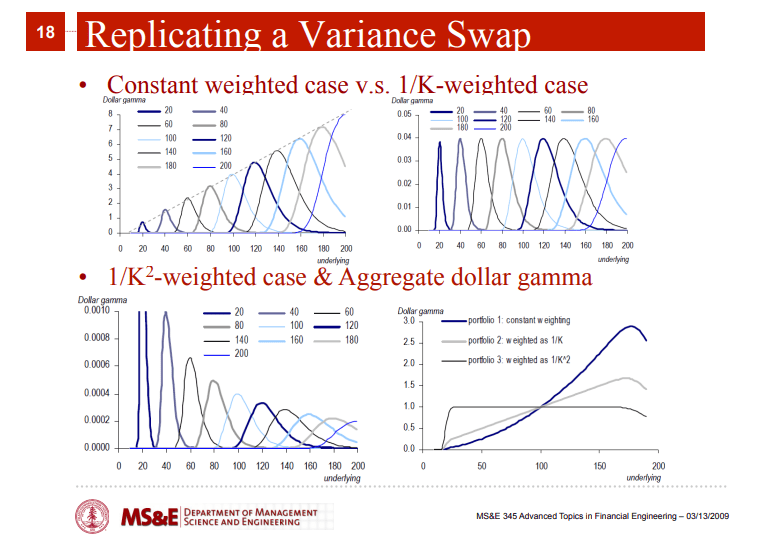Note that the common 1/K² weighting means variance swap pricing is highly sensitive to skew since the hedger’s portfolio weights downside puts so heavily. This is also why the strike of variance swaps can be much higher than the ATM vol of the same term. It reflects the cost of having constant gamma even as the market sells off. That is expensive because it requires owning beefy, sought-after low delta puts.

How Gamma Scales With Price, Volatility, and Time

Having an intuition for how gamma scales is useful when projecting how your portfolio will behave as market conditions or parameters change. A great way to get a feel for this is to tinker with an option calculator.  To demonstrate the effects of time, vol, and price, we hold 2 of the 3 constant and vary the 3rd.

Assume the strike is ATM for each example.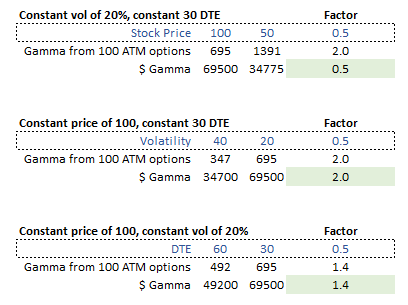Here are a few rules of thumb for how price, vol, and time affect gamma.

• If one ATM price is 1/2 the other, the lower price will also have 1/2 the dollar gamma. Linear effect as the higher gamma per option is offset by the dollar gamma’s extra weight to higher-priced stocks.
• If one ATM volatility is 1/2 the other, the dollar gamma is inversely proportional to the ratio of the vols (ie 1/vol ratio).
• If an option has 1/2 as much time until expiration, it will have √ratio of more gamma.

Stated differently:

• Spot prices have linearly proportional differences in gamma. The lower price has less dollar gamma.
• Volatility has inverse proportionality in gamma. The higher vol has less dollar gamma.
• Time is inversely proportional to gamma with a square root scaling. More time means less dollar gamma.

As you weight relative value trades these heuristics are handy (it’s also the type of things interview test your intuition for).

Some considerations that pop out if you choose to run a gamma-neutral book?

• Time spreads are tricky. You need to overweight the deferred months and since vega is positively proportional to root time, you will have large net vega exposures if you try to trade term structure gamma-neutral.
• Stocks with different vols. You need to overweight the higher vol stocks to be vega-neutral, but their higher volatility comes with higher theta. Your gamma-neutral position will have an unbalanced theta profile. This will be the case for inter-asset vol spreads but also intra-asset. Think of risk reversals that have large vol differences between the strikes.
• Overweighting lower-priced stocks to maintain gamma neutrality does not tend to create large imbalances because spot prices are positively proportional to other greeks (higher spot –> higher vega, higher dollar gamma, higher theta all else equal).

Weighting trades can be a difficult topic. How you weight trades really depends on what convergence you are betting on. If you believe vols move as a fixed spread against each other then you can vega-weight trades. If you believe vols move relative to each other (ie fixed ratio — all vols double together) then you’d prefer theta weighting.

I’ve summarized some of Colin Bennett’s discussion on weighting here. The context is dispersion, but the intuitions hold.

Finally, this is an example of a top-of-funnel tool to spot interesting surfaces. The notations on it tie in nicely with the topic of weightings. The data is ancient and besides the point.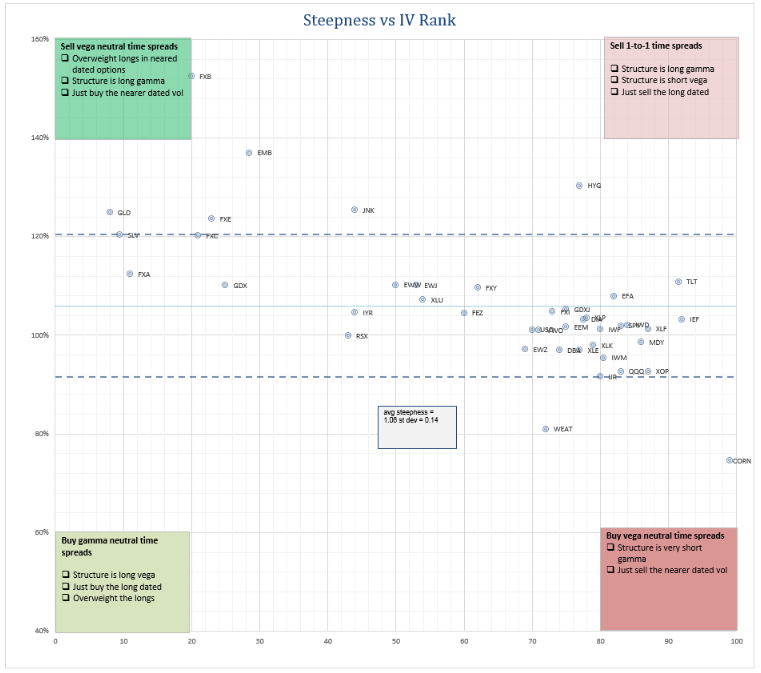# Wrapping Up

Gamma is the first higher-order greek people are exposed to. Like most of my posts, I try to approach it intuitively. I have always felt velocity and acceleration are the easiest bridges to understanding p/l curvature. While the first half of the post is intended for a broad audience, the second half is likely to advanced for novices and too rudimentary for veterans. If it helps novices who are trying to break into the professional world, I’ll consider that a win. I should add that in Finding Vol Convexity I apply the concept of convexity to implied volatility. You can think of that as the “gamma of vega”. In other words, how does an option’s vega change as volatility changes?

I realize I wrote that post which is more advanced than this one in the wrong order. Shrug.

1. Variance swap p/l is actually proportional to the difference in variance between the vol it was struck at and the realized variance. The payoff is therefore quadratic with respect to the spread of realized variance. Vol swaps pay off linearly with respect to the volatility spread, but since they are difficult to hedge (the option strip needs to be rebalanced where the variance swap has a “static hedge”, in that you do not need to rebalance the options portfolio, just the daily delta adjustment

## One thought on “Moontower on Gamma”

1.jitensaysjiten says:

Excellent post. If I have a PE short giving +Delta and CE short giving -Delta and the underlying moves higher, should I still look at position’s overall Gamma to determine my PnL. How will that be impacted because of Gamma closer to expiry?